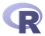# DATA SCIENCE COURSES

Each path is a curated sequence of courses, carefully arranged to take you from zero to job-ready.### Data Analyst – R

Beginner

You’ll learn the basics of using R for data analytics, how to work with data sources, data cleaning techniques, how to perform statistical analyses, data visualization techniques, and predictive analysis.

### Data Analyst – Python

Beginner

You’ll learn the basics of using Python for data analytics, how to work with data sources, data cleaning techniques, how to perform statistical analyses, data visualization techniques, and predictive analysis.

### Data Scientist – Python

Intermediate

You’ll learn advanced techniques for using Python for data analytics including: how to work with larger datasets, machine learning topics, and the basics of computer architecture and parallel processing.

### Data Engineer

You’ll learn how to work with production databases, how to build data pipelines that can handle larger data sets, and learn key computer science concepts like data structures, algorithms, and recursion.

## Browse Data Science Course Catalogue

Choose the courses that meet your specific needs. Each of our hands on courses allow you learn through coding so that you pick up concepts quickly.

### Python for Data Science: Fundamentals

Learn the basics of Python programming and data science.

FREE

### Python for Data Science: Intermediate

Learn the basics of Python programming and data science.

FREE

### Pandas & NumPy Fundamentals

Learn how to analyze data using the pandas and NumPy libraries.

FREE + BASIC

### Exploratory Data Visualization

Learn how to explore data by creating and interpreting data graphics. This course is taught using matplotlib and pandas.

BASIC

### Storytelling Through Data Visualization

Learn how to communicate insights and tell stories using data visualization.

BASIC

### Data Cleaning and Analysis

Learn how to clean and combine datasets, then practice your skills.

BASIC

### Data Cleaning Project Walkthrough

Learn how to clean and combine datasets, then practice your skills.

BASIC

### Command Line: Beginner

Learn the basics of the command line, a critical part of any data science workflow.

BASIC

### Command Line: Intermediate

Learn more about the command line and how to use it in your data analysis workflow.

BASIC

### Git & Version Control

Learn the basics of Python programming and data science.

BASIC

### SQL Fundamentals

Learn the basics of working with SQL databases.

BASIC

### SQL Intermediate: Table Relations & Joins

Learn to work with multi-table databases.

BASIC

### SQL & Databases: Advanced

Learn how to work with PostgreSQL, customize databases using indexing and how to improve database performance.

BASIC

### APIs & Web Scraping

Learn how to acquire data from APIs and the web.

BASIC

### Statistics: Fundamentals

Learn about sampling, variables and distributions.

BASIC

### Statistics Intermediate: Averages & Variability

Learn to summarize distributions, measure variability using variance or standard deviation, and compare values using z-scores.

BASIC

### Probability & Statistics in Python: Intermediate

Learn more advanced statistical concepts for more powerful data analysis.

BASIC

### Machine Learning Fundamentals

Learn the fundamentals of machine learning using k-nearest neighbors.

### Calculus for Machine Learning

Learn the calculus necessary for intermediate machine learning techniques like linear regression.

### Linear Algebra for Machine Learning

Learn the linear algebra necessary for intermediate machine learning techniques like linear regression.

### Linear Regression for Machine Learning

Learn how to use the linear regression machine learning model.

### Machine Learning in Python: Intermediate

Dive more into Machine learning.

### Decision Trees

Learn how to construct and interpret decision trees.

### Deep Learning: Fundamentals

Learn the basics of deep neural networks. Includes graph representation, activation functions, multiple hidden layers, and image classification.

### Machine Learning Project

Learn what a complete data science project looks like, from data cleaning to machine learning.

### Data Structures & Algorithms

Learn how computers work and how they work with data.

### Python Programming Advanced

Learn advanced concepts in Python, including more on object-oriented programming, lambda functions, and exception handling.

### Kaggle Fundamentals

Learn how to get started with and participate in Kaggle competitions with Kaggle's 'Titanic' competition.

### Exploring Topics in Data Science

Explore other topics in data science, like NLP and clustering.

### Natural Language Processing

Learn how to analyze and make predictions on textual data.

### Spark & Map-Reduce

Learn how to use Apache Spark and the map-reduce technique to clean and analyze large datasets.

### Postgres for Data Engineers

Learn about the SQL database Postgres.

### Optimizing Postgres Databases

Learn how to optimize your Postgres databases.

### Processing Large Datasets in Pandas

Learn how to work with datasets by optimizing your pandas workflow, processing data in batches, and augmenting pandas with SQLite.

### Optimizing Code Performance on Large Datasets

Learn how to process data more quickly by optimizing CPU and I/O performance. Learn to parallelize your code for better performance.

### Algorithms & Data Structures

Learn about different data structures, and how they can help speed up your data analysis.

### Recursion & Trees

Learn about recursion and how it applies to tree data structures, and how tree data structures are used to speed up processing of data analysis tasks.

### Building a Data Pipeline

Learn how to build a Python data pipeline from scratch.

### Introduction to Programming in R

Learn the basics of R, a popular programming language for data analysis.

FREE

### Intermediate R Programming

Enhance your data analysis skills in R.

FREE

### Data Visualization in R

Learn to use the ggplot2 package for exploratory data visualization in R.

FREE

### Data Cleaning in R

Learn to use the ggplot2 package for exploratory data visualization in R.

FREE